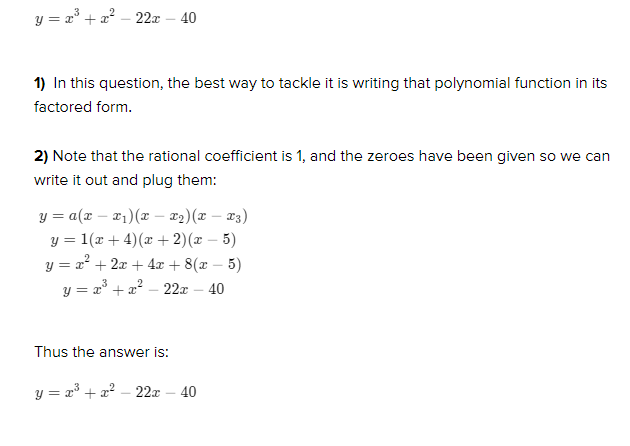# write the polynomial function f of least degree that hadsnajat3l 2022-02-11 Answered
write the polynomial function f of least degree that had rational coefficients of 1, and the given zeros. write the function in standard form. -4, -2,5
You can still ask an expert for help

• Questions are typically answered in as fast as 30 minutes

Solve your problem for the price of one coffee

• Math expert for every subject
• Pay only if we can solve itJustice Jacobson
Here is explanation###### Not exactly what you’re looking for?Cenedesiidv
y=a(x-x_1)(x-x_2)(x-x_3)
y=1(x+4)(x+2)(x-5)
y=x^2+2x+4x+8(x-5)
y=x^3+x^2-22x-40